# Using the Power Law Model to Quantify Shear Thinning Behavior on a Rotational Rheometer

A material’s rheological properties not only influences its visual and textural perception, but also affects its processing capabilities. For instance, compared to Newtonian materials, shear-thinning materials are more susceptible to applied stress. It is necessary to measure shear thinning behavior as most materials of interest come under this class of materials.

## Power Law Model

The power law region of a flow curve can be calculated to measure such behavior (Figure 1). This region shows linearity with a constant gradient on a log-log plot of viscosity vs. shear rate, but exhibits power law dependence on a linear scale plot.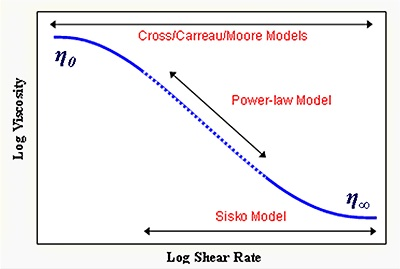Figure 1. Diagram showing an ideal flow curve and the relevant models for describing its shape.

The Power Law or Ostwald de Waele Model (Equation 1) can be used to mathematically express the power law region of a flow curve as follows: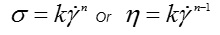Where, k = consistency; n = power law index; σ = shear rate; γ = shear rate consistency.

The range of power law index varies from ‘0’ for very shear thinning materials to ‘1’ for Newtonian materials. With the known values of other parameters, the viscosity can be estimated at any shear rate within the shear-thinning region. Nevertheless, the equation should not be used outside of the measured range of shear rates due to possibility of the presence of Newtonian region on either side of the measurement region, depending on the material being tested.

## Experimental Procedure

A table of shear rate test was performed and the resultant curve was analyzed by fitting a power law model in order to evaluate a skin lotion’s shear thinning behavior. With standard pre-configured sequences in the rSpace software, a Kinexus rotational rheometer in combination with a roughened parallel plate measuring system and a Peltier plate cartridge was employed to make rotational rheology measurements at 25°C. The use of a standard loading sequence ensures that both samples had undergone a constant and controllable loading protocol. An equilibrium table of shear rates test was used to generate the flow curve between 0.1 and 100 s-1. A portion of this curve was manually selected and fitted with a power law model.

## Experimental Results

The viscosity-shear rate curve for the sample is depicted in Figure 2, showing shear thinning behavior with a sudden drop in viscosity against increasing shear rate. A slight curvature is observed at higher shear rates and the data shows linearity at lower shear rates on a double-logarithmic plot. Hence, the analysis has taken only the data between 0.1 and 10s-1 that shows linearity in this region on a logarithmic plot.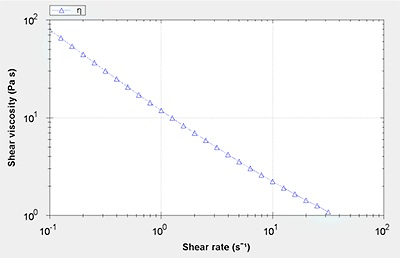Figure 2. Viscosity-shear rate data for a skin lotion.

Figure 3 shows both the original data and the power law model fitted data, and Table 1 summarizes the fitting parameters and correlation coefficient.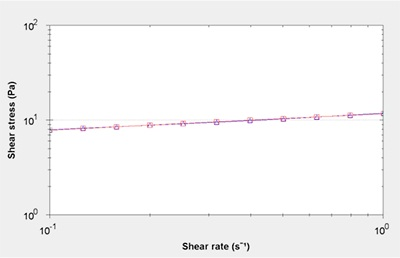Figure 3. Original data (blue) and power law model fitted data (red) between 0.1 and 10s-1.

Table 1. Model fitting parameter data.

Sample Description Experiment Name Action Name k1 n Chi square Correlation coefficient
Skin Lotion Analyse_0004-1 Power Law model fit 11.71 0.1735 617.2 0.9908

The results show that the sample is highly shear thinning, if shear thinning index assigned for Newtonian materials and non-Newtonian materials is ‘1’ and ‘0,’ respectively. Many models require this shear thinning index to describe the flow behavior of non-Newtonian liquids. The numerically equal value of the consistency ‘k’ is the viscosity at 1s-1.

For this sample, k value is 11.71, which can be used for viscosity measurements for comparative purposes. The correlation coefficient value of the sample is 0.988, showing good agreement between predicted and measured data. Similar data and the corresponding fitted data for other consumer products are depicted in Figure 4.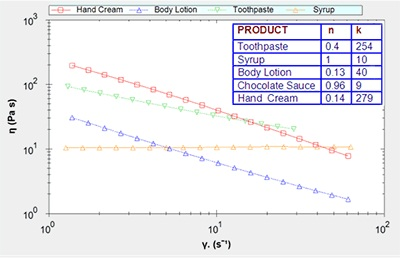Figure 4. Data and power law model fits for various samples.

With the known ‘n’ and ‘k’ values, the viscosity can be predicted at any shear rate by applying the power law equation. This resulting values are useful in the selection of optimum packaging, reformulation of a product to satisfy specific needs, or determination of the shear thinning behavior of the product on the packing line or during production. However, it is important to use this model to predict shear thinning behavior within the power law region. The Sisko or Cross models can be used to determine shear thinning behavior outside of this region.

## Conclusion

This article described the evaluation of a skin lotion’s shear thinning behavior by carrying out a table of shear rate test and subsequent analysis of the resultant curve with a power law model, which yielded a good fit to the flow curve between the values 0.1 and 10s-1. The results revealed that the sample studied is a highly shear thinning and has a viscosity 1000 folds higher than water at 1s-1 shear rate. The results demonstrate the applicability of the power law model to quantify shear thinning behavior and to compare different formulations and products.This information has been sourced, reviewed and adapted from materials provided by Malvern Panalytical.

## Citations

• APA

Malvern Panalytical. (2019, September 03). Using the Power Law Model to Quantify Shear Thinning Behavior on a Rotational Rheometer. AZoM. Retrieved on February 18, 2020 from https://www.azom.com/article.aspx?ArticleID=11624.

• MLA

Malvern Panalytical. "Using the Power Law Model to Quantify Shear Thinning Behavior on a Rotational Rheometer". AZoM. 18 February 2020. <https://www.azom.com/article.aspx?ArticleID=11624>.

• Chicago

Malvern Panalytical. "Using the Power Law Model to Quantify Shear Thinning Behavior on a Rotational Rheometer". AZoM. https://www.azom.com/article.aspx?ArticleID=11624. (accessed February 18, 2020).

• Harvard

Malvern Panalytical. 2019. Using the Power Law Model to Quantify Shear Thinning Behavior on a Rotational Rheometer. AZoM, viewed 18 February 2020, https://www.azom.com/article.aspx?ArticleID=11624.

1.Ümit Nazlı Temel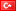says:

Hi,
Did yo use rheology software for curve fitting.

The opinions expressed here are the views of the writer and do not necessarily reflect the views and opinions of AZoM.com.